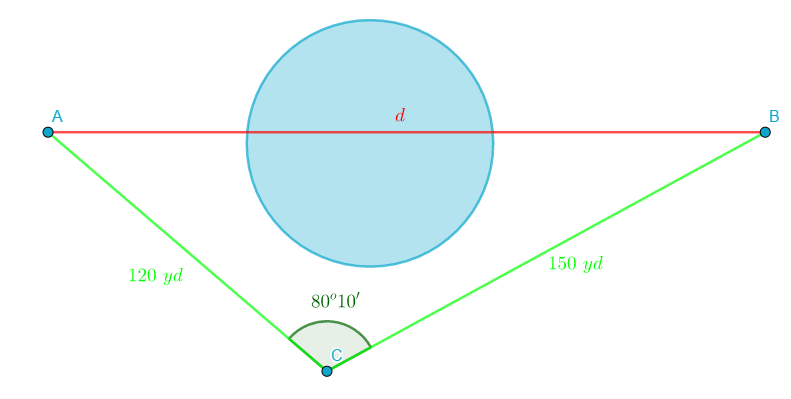### Math Notes

Subjects

#### Trigonometry Solutions

##### Topics || Problems

Points $$A$$ and $$B$$ are separated by an obstacle. In order to find the distance between them, a third point $$C$$ is selected which is $$120 ~ yards$$ from $$A$$ and $$150~yards$$ from $$B$$. The angle $$ABC$$ is measured to be $$80^o10'$$. Find the distance from $$A$$ to $$B$$.Let the distance between $$A$$ and $$B$$ be $$d$$

By cosine law, the value of $$d$$ can be calculated.

Thus,

$$d^2 = 150^2 + 120^2 -2(150)(120)\cos{80^o10'}$$

$$d^2 = 30751.82$$

$$d = \sqrt{30751.82}$$

$$d \approx 175.36 ~yards$$